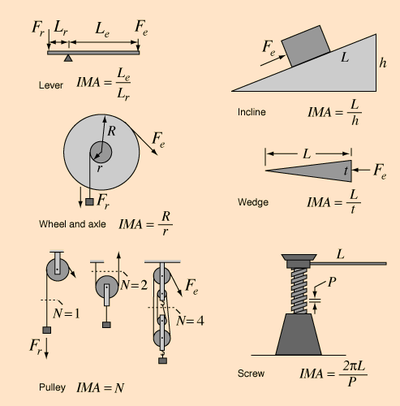Figure 1: Types of simple machines and their corresponding mechanical advantages. Mechanical advantages allows humans to perform tasks much easier in terms of the force they need to apply, but must always obey the conservation of energy.

Mechanical advantage is a measure of the ratio of output force to input force in a system, used to analyze the forces in simple machines like levers and pulleys. Despite changing the forces that are applied the conservation of energy is still true and the output energy is still equal to the input energy. Typically the mechanical advantage is expressed in ideal terms, where there is no losses in energy between the input and output times, also known as 100% efficient systems.

By conservation of energy:

The left side is the input energy (or work put into a machine), and the right side is the output energy (or work that comes out of a machine, for no-loss energy exchange). Rearranging the equation gives the ideal mechanical advantage for a system, expressed as:

This can be applied to many physical situations with rigid bodies, and can be used to analyze the force advantage gained in using various tools. Simple machines use mechanical advantage as a key property to their functionality, helping humans perform tasks that would be require more force than a person could produce. Even work animals (like horses or oxen) and engines benefit from using the mechanical advantage of simple machines.